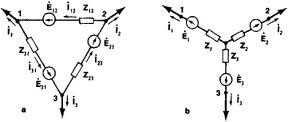## From the Delta Connection Assignment Help

Assignment Help: >> Conversion from Star Connection to Delta Connection - From the Delta Connection

From the Delta Connection:

Resistance among terminals 1 and 2 whereas 3 is open circuited.

Rc and Rb are in series. This combination is in parallel to Ra.

R12 =  Ra (Rb + Rc ) / Ra  + Rb + Rc

Figure 4.51

Likewise, the resistances between terminals 2 and 3 and 1 and 3 are given by :

R23= (Ra  + Rc ) Rb/ Ra  + Rb + Rc

R13 = (Ra  + Rb ) Rc / Ra  + Rb + Rc

Now compare the results of star and delta connections:

R 1 + R2 = Ra (Rb + Rc )/ Ra  + Rb + Rc

R 2  + R3  =  Rb (Ra  + Rc )/ Ra  + Rb + Rc

R 1 + R3 = Rc (Ra + Rb )/ Ra + Rb + Rc

We may determine three unknown resistances Ra, Rb and Rc in terms of R1, R2 and R3.

R a   = R1 R2 + R2 R3 + R3 R1/ R3

R b   = R1 R2 + R2 R3 + R3 R1/ R1

R c  = R1 R2 + R2 R3 + R3 R1 / R2